## D3.js calendar configuration in OBIEE 11g

With the
release of the 11.1.1.6.2 BP1 SampleApp V207, I’ve taken a moment to dissect
the usage of the D3.js calendar contained within since it is one of the
examples frequently pointed out to showcase new visualisation capabilities.
Let’s dive a bit deeper into this to understand the way the D3 calendar works and what is necessary to embed this
in any other OBIEE installation.
As-Is in
SampleApp: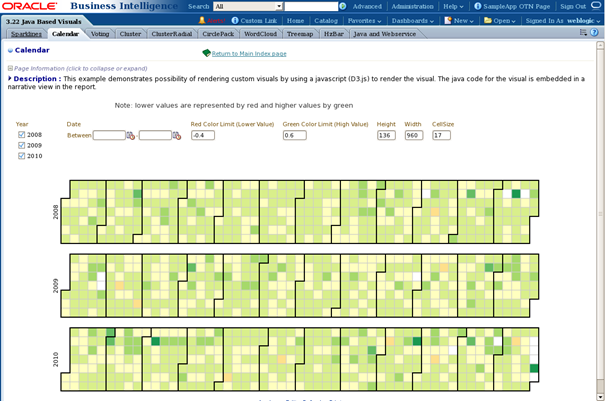Checking
the Answers Criteria block, the analysis is quite straight-forward. “Color Low”
and “Color High” being simply presentation variables grabbing the values passed
from the “Red / Green Color Limit” prompts on the dashboard page.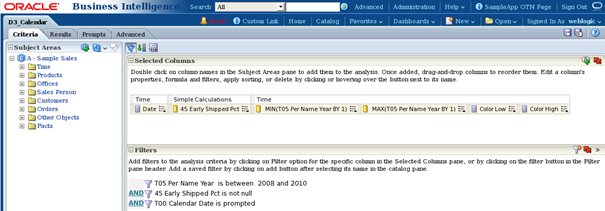The actual
functionality of the D3 calendar is contained and controlled by the two narrative
views of the analysis which I will be calling the “Context Narrative” and “Content
Narrative” respectively for the purpose of this post: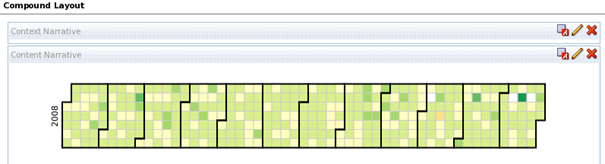Context
narrative: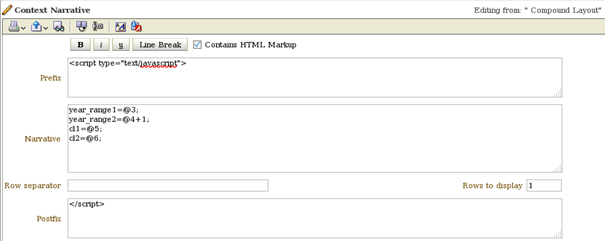Key here are
the four JavaScript variables which will be used by the code within the content
narrative below. These are filled by the values collected from the 1st
line of the analysis data set (Rows to display = 1). Generally, when analysing
the D3.js examples, it is hugely helpful to add a simple table view to the
analysis in order to see what the data stream actually brings along from the OBI
server since most examples use mixtures of column references and variables.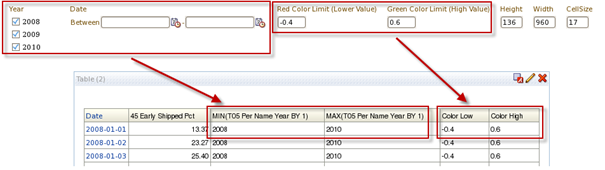VERY
IMPORTANT: year_range2 must always be the end year +1!
You don’t
have to provide these values through dashboard prompts and simply read the from
the data set, but having a means to restrict the data displayed is always comfortable.

Content
narrative: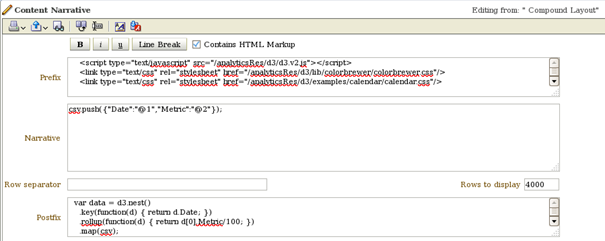Prefix:

<script type=”text/javascript” src=”/analyticsRes/d3/d3.v2.js”></script>

<div id=”my_chart”></div>
<script type=”text/javascript”>
var margin = {top: 19, right: 20, bottom: 20, left: 19},
width = @{Width}{720}- margin.right – margin.left, // width
height = @{Height}{136} – margin.top – margin.bottom, // height
cellSize = @{CellSize}{12}; // cell size

var day = d3.time.format(“%w”),
week = d3.time.format(“%U”),
percent = d3.format(“.1%”),
format = d3.time.format(“%Y-%m-%d”);

var color = d3.scale.quantize()
.domain([cl1,cl2])
.range(d3.range(9));

var svg = d3.select(“#my_chart”).selectAll(“svg”)
.data(d3.range(year_range1, year_range2))
.enter().append(“svg”)
.attr(“width”, width + margin.right + margin.left)
.attr(“height”, height + margin.top + margin.bottom)
.attr(“class”, “RdYlGn”)
.append(“g”)
.attr(“transform”, “translate(” + (margin.left + (width – cellSize * 53) / 2) + “,” + (margin.top + (height – cellSize * 7) / 2) + “)”);
svg.append(“text”)
.attr(“transform”, “translate(-6,” + cellSize * 3.5 + “)rotate(-90)”)
.attr(“text-anchor”, “middle”)
.text(String);
var rect = svg.selectAll(“rect.day”)
.data(function(d) { return d3.time.days(new Date(d, 0, 1), new Date(d + 1, 0, 1)); })
.enter().append(“rect”)
.attr(“class”, “day”)
.attr(“width”, cellSize)
.attr(“height”, cellSize)
.attr(“x”, function(d) { return week(d) * cellSize; })
.attr(“y”, function(d) { return day(d) * cellSize; })
.datum(format);
rect.append(“title”)
.text(function(d) { return d; });
svg.selectAll(“path.month”)
.data(function(d) { return d3.time.months(new Date(d, 0, 1), new Date(d + 1, 0, 1)); })
.enter().append(“path”)
.attr(“class”, “month”)
.attr(“d”, monthPath);

var csv =[];

What happens
here that I can influence?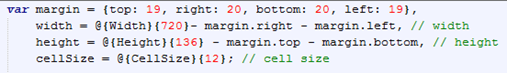This
controls the size of the rendered calendar. Parameters are passed from the dashboard
prompt via presentation variables. If you don’t want users to be able to choose
this, simply put static values.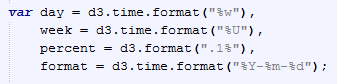Date format
is controlled here. VERY IMPORTANT: you must match the date format in this part
of the script to the date format provided within the data set: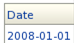means “format
= d3.time.format(“%Y-%m-%d”);”
If your
date picture is for example DD.MM.YYYY (e.g. 14.08.2012), then you need to
format = d3.time.format(“%d.%m.%Y “);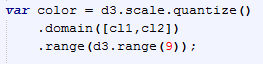Specifies
the colour limits (.domain) as retrieved by the dashboard prompts.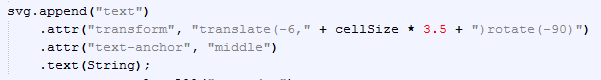Controls
the display of the year number in front of the rectangles representing the
year: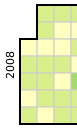Leave the
rest as-is.
Narrative:
csv.push({“Date”:”@1″,”Metric”:”@2″});
Simply
retrieves the values for date and measure from the data set (per row!). Leave
this as-is.
Postfix:
var data = d3.nest()
.key(function(d) { return d.Date; })
.rollup(function(d) { return d.Metric/100; })
.map(csv);

rect.filter(function(d) { return d in data; })
.attr(“class”, function(d) { return “day q” + color(data[d]) + “-9”; })
.select(“title”)
.text(function(d) { return d + “: ” + percent(data[d]); });

function monthPath(t0) {
var t1 = new Date(t0.getFullYear(), t0.getMonth() + 1, 0),
d0 = +day(t0), w0 = +week(t0),
d1 = +day(t1), w1 = +week(t1);
return “M” + (w0 + 1) * cellSize + “,” + d0 * cellSize
+ “H” + w0 * cellSize + “V” + 7 * cellSize
+ “H” + w1 * cellSize + “V” + (d1 + 1) * cellSize
+ “H” + (w1 + 1) * cellSize + “V” + 0
+ “H” + (w0 + 1) * cellSize + “Z”;
}
</script>

What happens
here that I can influence?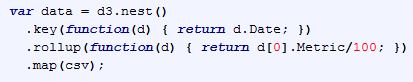For the SampleApp
example, this transforms the values retrieved from the data set into an index representation
for transformation into a percentile representation in the tooltip display.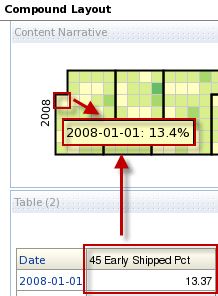If you want absolute
values, simply change
“.rollup(function(d) {
return d.Metric/100; })”
to
“.rollup(function(d) {
return d.Metric; })”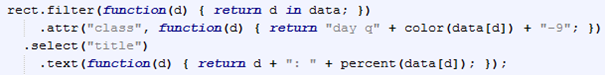This colours the date
rectangles and the actual tooltip with date information and the added percentile
representation of the measure. Here, if you want absolute values, simply change
“.text(function(d) {
return d + “: ” + percent(data[d]); });”
to
“.text(function(d) {
return d + “: ” + data[d] });”
Leave the rest as-is.
So what do you need
all-in-all to transplant this into any OBIEE implementation?

1.) The
D3 libraries contained in the d3 and d3-cloud folders. Quickest way – if you
have the SampleApp lying around – is to just grab them from the analyticsRes
folder of the VirtualBox and slap them into your own analyticsRes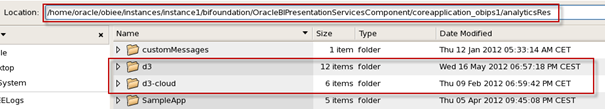2.) An
analysis on the date (day!) grain with some measure. Since you can either
hard-code the time ranges, size and colour limits, those two are really the
bare minimum for this D3 calendar to work.
That’s it. Bob’s your
uncle.

UPDATE: Since the D3.js libraries are only dependent of the final browser interpreting the generated code (IE8 will NOT work!), you can transplant this into any version of OBIEE that you fancy. This functionality is NOT tied to OBIEE 11.1.1.6.